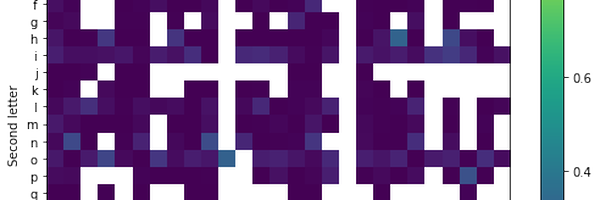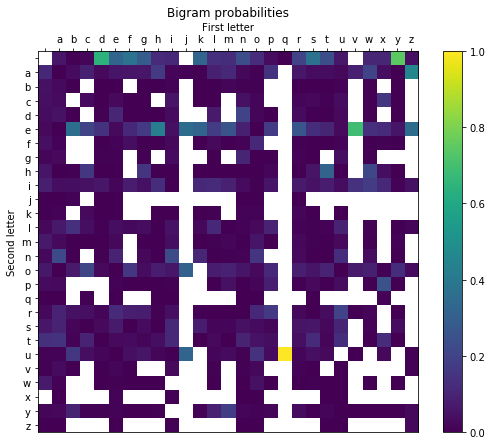# Solving substitution ciphers with Markov chains in Python

A simple substitution cipher like a Caesar cipher or ROT13 substitutes each letter in the original message with a specific letter, e.g. replacing all A's in the original message with N's. So a message like:

TO BE OR NOT TO BE

becomes:

LW UO WQ PWL LW UO

We can break these ciphers using some basic natural language processing, exploiting statistical properties of language. If you know some basic cryptography you might be familiar with the idea of using letter frequencies for this kind of code-breaking, e.g. the fact that 'e' is the most common letter in English. We'll use letter frequencies here, but we'll also add bigram frequencies (pairs of letters), as used in Chen and Rosenthal (2012).

By using bigram frequencies to evaluate the likelihood that text belongs to English, we're assuming that it resembles a Markov process - when `c1` and `c2` appear together, we only condition on `c1` to get an estimate of how likely `c2` was to appear, rather than the entire text up to that point. Since we're only considering one previous letter, it's a first-order Markov process.

## Getting some text (or maybe a lot)

We'll start by setting up some sources of text, both to encrypt/decrypt and to estimate the letter and bigram frequencies from. For both uses, it helps to have reasonably large amounts of text (while testing this method I found it didn't always work well on short cipher texts). We'll use some books chosen from the most popular downloads on Project Gutenberg.

First let's get some basic libraries and functions set up:

``````import collections
from itertools import islice
import math
import random
import string

import pandas as pd
import matplotlib.pyplot as plt

# Helper function we'll use to preview dictionaries
def take(n, iterable):
return list(islice(iterable, n))

# Get all the text from a file, converting to lowercase
#   and removing everything except letters and spaces
def get_simple_text(filename):
with open(filename) as file_in:
# Remove all punctuation + numbers
out = []
for c in all_text.lower():
# Make sure we don't add double spaces
if c in (' ', '\n', '\t', '\r'):
if out and out[-1] != ' ':
out.append(' ')
if c in string.ascii_lowercase:
out.append(c)
return ''.join(out)
``````

Now we'll read in our two books: Alice's Adventures in Wonderland and Frankenstein.

``````alice = get_simple_text('alice.txt')
frank = get_simple_text('frank.txt')
print(alice[1012:1105])
print(frank[3000:3100])
``````
``````the hot day made her feel very sleepy and stupid whether the pleasure of making a daisychain
rks in a little boat with his holiday mates on an expedition of discovery up his native river but su
``````

After that, it's time to set up an encryption key. We'll map each letter in the alphabet randomly to another letter:

``````def make_random_key():
out_letters = list(string.ascii_lowercase)
random.shuffle(out_letters)
key = dict(zip(string.ascii_lowercase, out_letters))
return key

encrypt_key = make_random_key()
take(5, encrypt_key.items())
``````
``````[('a', 'b'), ('b', 'a'), ('c', 'c'), ('d', 'f'), ('e', 'u')]
``````

And we'll encrypt Alice's Adventures in Wonderland:

``````# encrypting and decrypting both work the same
#   way except the key is reversed, so we won't
#   define a separate encrypt method
def decrypt(code, key):
trans = str.maketrans(key)
return code.translate(trans)

alice_encrypted = decrypt(alice, encrypt_key)
alice_encrypted[1012:1105]
``````
``````'wlu lpw fbn ibfu luy juuh suyn xhuutn bvf xwztof kluwluy wlu thubxzyu pj ibeovq b fboxnclbov '
``````

## The probability of a string

Now we'll use Frankenstein to estimate English letter and bigram probabilities.

For letter probabilities, all we have to do is count the number of times each letter appears, and divide by the total to convert into a probability.

For bigram probabilities, for each letter `c1`, we count all the letters that immediately follow the letter `c2`, and then convert to a probability `p(c1, c2) = count(c1, c2) / total(c1)`.

``````def make_letter_probs(text):
counts = collections.Counter(text)
total = sum(counts.values())

probs = {}
for c in counts:
# Ignore space
if c == ' ':
continue
probs[c] = counts[c] / total
return probs

def make_bigram_probs(text):
freqs = collections.defaultdict(collections.Counter)
for c1, c2 in zip(text[:-1], text[1:]):
freqs[c1][c2] += 1

prob_table = collections.defaultdict(dict)
for c1, c1_counts in freqs.items():
total = sum(c1_counts.values())
for c2, freq in c1_counts.items():
prob_table[c1][c2] = freq / total
return prob_table
``````
``````letter_probs = make_letter_probs(frank)
take(5, letter_probs.items())
``````
``````[('p', 0.014430786931051081),
('r', 0.048974512497211756),
('o', 0.059235257516524024),
('j', 0.0011833902722502025),
('e', 0.1081557660925815)]
``````
``````bigram_probs = make_bigram_probs(frank)
take(5, bigram_probs['a'].items())
``````
``````[('n', 0.21939004335476156),
('r', 0.09900583046793243),
('f', 0.009904320526237105),
('t', 0.14654656899387053),
('l', 0.06701300642846464)]
``````

We can use a quick plot to check how these bigram probabilities look. You can see some basic patterns, like how 'q' is almost always followed by 'u', and letters like 'v' and 'j' are mostly followed by vowels:

``````bi_df = pd.DataFrame.from_dict(bigram_probs)
bi_df.sort_index(axis = 'columns', inplace=True)

fig, ax = plt.subplots()
im = ax.imshow(bi_df, cmap=plt.cm.viridis, vmin=0, vmax=1)
fig.colorbar(im)
ax.set_title("Bigram probabilities")
ax.xaxis.tick_top()
ax.set_xlabel('First letter')
ax.set_ylabel('Second letter')
ax.xaxis.set_label_position('top')
ax.set_xticks(range(27))
ax.set_yticks(range(27))
ax.set_xticklabels(' ' + string.ascii_lowercase)
ax.set_yticklabels(' ' + string.ascii_lowercase)
fig.set_size_inches((9, 7))
``````We'll use our letter and bigram probabilities to score the likelihood of a piece of text being English (at least, English as it appears in Frankenstein). For each letter in the text we're scoring, we'll add the letter and bigram probability together (with optional weights). The overall likelihood would be the product of all these probabilities, which would get incredibly small very quickly, so instead we use the sum of the log probabilities.

The actual likelihood value we see is pretty arbitrary, the important thing is that the bigger it is, the more likely the text is to be English.

``````def score_text(text, letter_probs, bigram_probs,
letter_weight=1.0,
bigram_weight=1.0):
# Normalise weights to sum to 1
total_weight = letter_weight + bigram_weight
letter_weight = letter_weight / total_weight
bigram_weight = bigram_weight / total_weight

total_logprob = 0
for c1, c2 in zip(text[:-1], text[1:]):
# Use a default of 1 for letter prob, basically
#   ignore spaces
letter_prob = letter_probs.get(c1, 1)
bigram_prob = bigram_probs[c1].get(c2, 0.001)
total_logprob += math.log(
letter_weight * letter_prob +
bigram_weight * bigram_prob
)

score_text(alice_encrypted, letter_probs, bigram_probs)
``````
``````-510545.2674804321
``````

## Solving the cipher

With all these pieces in place, we can start trying to decrypt the text. We'll start with a random decryption key, and randomly swap letters around to see if we get an improvement in the decrypted text's score.

As we go we'll print out the current decryption of the text to see our progress. Even when we don't have the right answer, you should be able to see the text becoming more "English-y" as we go:

``````letter_weight = 1.0
bigram_weight = 1.0
iterations = int(1e4)
print_every = 1000

decrypt_key = make_random_key()
best_decrypt = decrypt(alice_encrypted, decrypt_key)
best_score = score_text(best_decrypt, letter_probs, bigram_probs,
letter_weight = letter_weight,
bigram_weight = bigram_weight)

for iter_num in range(iterations):
a, b = random.choices(string.ascii_lowercase, k=2)
# Swap two letters
decrypt_key[a], decrypt_key[b] = decrypt_key[b], decrypt_key[a]
current_decrypt = decrypt(alice_encrypted, decrypt_key)
new_score = score_text(current_decrypt, letter_probs, bigram_probs,
letter_weight = letter_weight,
bigram_weight = bigram_weight)
if new_score > best_score:
best_score = new_score
else:
# Swap back
decrypt_key[a], decrypt_key[b] = decrypt_key[b], decrypt_key[a]
# Check progress
if iter_num % print_every == 0:
print('{n}: {d}'.format(n=iter_num,
d=current_decrypt[1012:1105]))
print(current_decrypt[1012:1105])
``````
``````0: phu hwp fay jafu hub suuo euby couuxy azf cpkxif dhuphub phu xouackbu ws janizg a faicythaiz
1000: the hot day zade her lees very fseepy and ftupid whether the pseafure ol zaking a daifychain
2000: the hot day made her feew very sweepy and stupid lhether the pweasure of making a daisychain
3000: the hot day made her leef very sfeepy and stupid whether the pfeasure ol making a daisychain
4000: ths hot day mads hsr fssl vsry elsspy and etupid whsthsr ths plsaeurs of making a daieychain
5000: the hot day made hej feel vejy sleepy and stupid whethej the pleasuje of making a daisychain
6000: thc hot day madc hcr fccl vcry slccpy and stupid whcthcr thc plcasurc of making a daisyehain
7000: the hot dak made her feel verk sleepk and stupid whether the pleasure of maying a daiskchain
8000: the hot day made her feel very sleepy and stupid whether the pleasure of making a daisychain
9000: the hot day nade her feel very sleepy amd stupid whether the pleasure of nakimg a daisychaim
the hot day made her feel very sleepy and stupcd whether the pleasure of makcng a dacsyihacn
``````

And that's it! This is a random process so it may not find the correct answer every time, and might even move away after finding the right answer, but it should be clear that it generally moves in the direction of "more like English". We can check our final answer against the original encryption key we used:

``````def reverse_key(key):
return {c2: c1 for c1, c2 in key.items()}

true_decrypt_key = reverse_key(encrypt_key)
decrypt_key == true_decrypt_key
``````
``````True
``````

Because this method is so simple, it's easy to see multiple different ways we can tweak it to try for better accuracy and efficiency:

• Varying the weights on letters vs. bigrams.
• Using multiple texts to build up our letter and bigram probabilities to avoid the quirks of any one text.
• Extending to include trigrams or even longer sequences and whole words.
• Testing exactly how much text is needed to get good performance: shorter cipher texts are quicker to decrypt and score, but may not give as good accuracy since their score will have greater variance.